# bezier.curve module¶

Helper for Bézier Curves.

See Curve-Curve Intersection for examples using the Curve class to find intersections.

class bezier.curve.Curve(nodes, degree, *, copy=True, verify=True)

Bases: bezier._base.Base

Represents a Bézier curve.

We take the traditional definition: a Bézier curve is a mapping from $$s \in \left[0, 1\right]$$ to convex combinations of points $$v_0, v_1, \ldots, v_n$$ in some vector space:

$B(s) = \sum_{j = 0}^n \binom{n}{j} s^j (1 - s)^{n - j} \cdot v_j$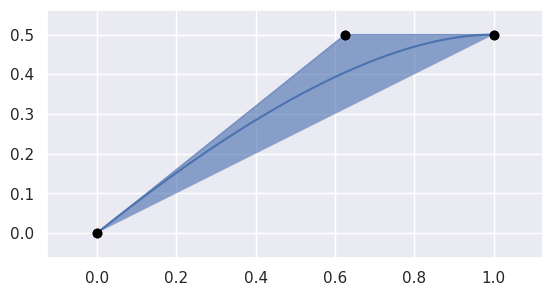>>> import bezier
>>> nodes = np.asfortranarray([
...     [0.0, 0.625, 1.0],
...     [0.0, 0.5  , 0.5],
... ])
>>> curve = bezier.Curve(nodes, degree=2)
>>> curve
<Curve (degree=2, dimension=2)>

Parameters
• nodes (Sequence [ Sequence [ numbers.Number ] ]) – The nodes in the curve. Must be convertible to a 2D NumPy array of floating point values, where the columns represent each node while the rows are the dimension of the ambient space.

• degree (int) – The degree of the curve. This is assumed to correctly correspond to the number of nodes. Use from_nodes() if the degree has not yet been computed.

• copy (bool) – Flag indicating if the nodes should be copied before being stored. Defaults to True since callers may freely mutate nodes after passing in.

• verify (bool) – Flag indicating if the degree should be verified against the number of nodes. Defaults to True.

classmethod from_nodes(nodes, copy=True)

Create a Curve from nodes.

Computes the degree based on the shape of nodes.

Parameters
Returns

The constructed curve.

Return type

Curve

property length

The length of the current curve.

Computes the length via:

$\int_{B\left(\left[0, 1\right]\right)} 1 \, d\mathbf{x} = \int_0^1 \left\lVert B'(s) \right\rVert_2 \, ds$
Returns

The length of the current curve.

Return type

float

copy()

Make a copy of the current curve.

Returns

Copy of current curve.

Return type

Curve

evaluate(s)

Evaluate $$B(s)$$ along the curve.

This method acts as a (partial) inverse to locate().

See evaluate_multi() for more details.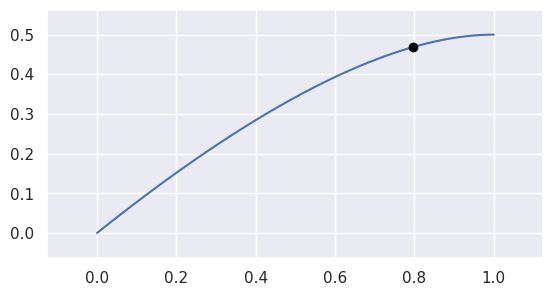>>> nodes = np.asfortranarray([
...     [0.0, 0.625, 1.0],
...     [0.0, 0.5  , 0.5],
... ])
>>> curve = bezier.Curve(nodes, degree=2)
>>> curve.evaluate(0.75)
array([[0.796875],
[0.46875 ]])

Parameters

s (float) – Parameter along the curve.

Returns

The point on the curve (as a two dimensional NumPy array with a single column).

Return type

numpy.ndarray

evaluate_multi(s_vals)

Evaluate $$B(s)$$ for multiple points along the curve.

This is done via a modified Horner’s method (vectorized for each s-value).

>>> nodes = np.asfortranarray([
...     [0.0, 1.0],
...     [0.0, 2.0],
...     [0.0, 3.0],
... ])
>>> curve = bezier.Curve(nodes, degree=1)
>>> curve
<Curve (degree=1, dimension=3)>
>>> s_vals = np.linspace(0.0, 1.0, 5)
>>> curve.evaluate_multi(s_vals)
array([[0.  , 0.25, 0.5 , 0.75, 1.  ],
[0.  , 0.5 , 1.  , 1.5 , 2.  ],
[0.  , 0.75, 1.5 , 2.25, 3.  ]])

Parameters

s_vals (numpy.ndarray) – Parameters along the curve (as a 1D array).

Returns

The points on the curve. As a two dimensional NumPy array, with the columns corresponding to each s value and the rows to the dimension.

Return type

numpy.ndarray

evaluate_hodograph(s)

Evaluate the tangent vector $$B'(s)$$ along the curve.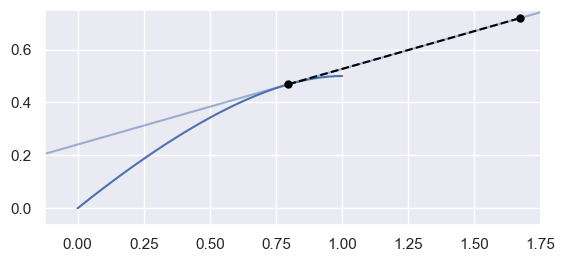>>> nodes = np.asfortranarray([
...     [0.0, 0.625, 1.0],
...     [0.0, 0.5  , 0.5],
... ])
>>> curve = bezier.Curve(nodes, degree=2)
>>> curve.evaluate_hodograph(0.75)
array([[0.875],
[0.25 ]])

Parameters

s (float) – Parameter along the curve.

Returns

The tangent vector along the curve (as a two dimensional NumPy array with a single column).

Return type

numpy.ndarray

plot(num_pts, color=None, alpha=None, ax=None)

Plot the current curve.

Parameters
Returns

The axis containing the plot. This may be a newly created axis.

Return type

matplotlib.artist.Artist

Raises

NotImplementedError – If the curve’s dimension is not 2.

subdivide()

Split the curve $$B(s)$$ into a left and right half.

Takes the interval $$\left[0, 1\right]$$ and splits the curve into $$B_1 = B\left(\left[0, \frac{1}{2}\right]\right)$$ and $$B_2 = B\left(\left[\frac{1}{2}, 1\right]\right)$$. In order to do this, also reparameterizes the curve, hence the resulting left and right halves have new nodes.>>> nodes = np.asfortranarray([
...     [0.0, 1.25, 2.0],
...     [0.0, 3.0 , 1.0],
... ])
>>> curve = bezier.Curve(nodes, degree=2)
>>> left, right = curve.subdivide()
>>> left.nodes
array([[0.   , 0.625, 1.125],
[0.   , 1.5  , 1.75 ]])
>>> right.nodes
array([[1.125, 1.625, 2.   ],
[1.75 , 2.   , 1.   ]])

Returns

The left and right sub-curves.

Return type
intersect(other, strategy=<IntersectionStrategy.GEOMETRIC: 0>, _verify=True)

Find the points of intersection with another curve.

See Curve-Curve Intersection for more details.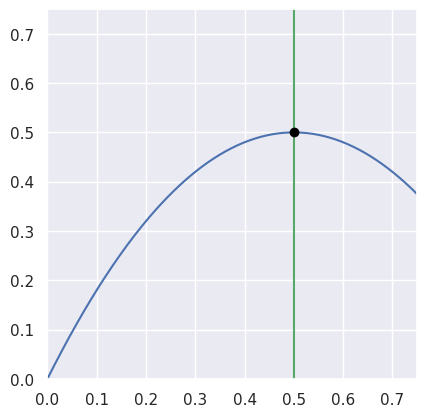>>> nodes1 = np.asfortranarray([
...     [0.0, 0.375, 0.75 ],
...     [0.0, 0.75 , 0.375],
... ])
>>> curve1 = bezier.Curve(nodes1, degree=2)
>>> nodes2 = np.asfortranarray([
...     [0.5, 0.5 ],
...     [0.0, 0.75],
... ])
>>> curve2 = bezier.Curve(nodes2, degree=1)
>>> intersections = curve1.intersect(curve2)
>>> 3.0 * intersections
array([[2.],
[2.]])
>>> s_vals = intersections[0, :]
>>> curve1.evaluate_multi(s_vals)
array([[0.5],
[0.5]])

Parameters
Returns

2 x N array of s- and t-parameters where intersections occur (possibly empty).

Return type

numpy.ndarray

Raises
elevate()

Return a degree-elevated version of the current curve.

Does this by converting the current nodes $$v_0, \ldots, v_n$$ to new nodes $$w_0, \ldots, w_{n + 1}$$ where

\begin{split}\begin{align*} w_0 &= v_0 \\ w_j &= \frac{j}{n + 1} v_{j - 1} + \frac{n + 1 - j}{n + 1} v_j \\ w_{n + 1} &= v_n \end{align*}\end{split}>>> nodes = np.asfortranarray([
...     [0.0, 1.5, 3.0],
...     [0.0, 1.5, 0.0],
... ])
>>> curve = bezier.Curve(nodes, degree=2)
>>> elevated = curve.elevate()
>>> elevated
<Curve (degree=3, dimension=2)>
>>> elevated.nodes
array([[0., 1., 2., 3.],
[0., 1., 1., 0.]])

Returns

The degree-elevated curve.

Return type

Curve

reduce_()

Return a degree-reduced version of the current curve.

Does this by converting the current nodes $$v_0, \ldots, v_n$$ to new nodes $$w_0, \ldots, w_{n - 1}$$ that correspond to reversing the elevate() process.

This uses the pseudo-inverse of the elevation matrix. For example when elevating from degree 2 to 3, the matrix $$E_2$$ is given by

$\begin{split}\mathbf{v} = \left[\begin{array}{c c c} v_0 & v_1 & v_2 \end{array}\right] \longmapsto \left[\begin{array}{c c c c} v_0 & \frac{v_0 + 2 v_1}{3} & \frac{2 v_1 + v_2}{3} & v_2 \end{array}\right] = \frac{1}{3} \mathbf{v} \left[\begin{array}{c c c c} 3 & 1 & 0 & 0 \\ 0 & 2 & 2 & 0 \\ 0 & 0 & 1 & 3 \end{array}\right]\end{split}$

and the (right) pseudo-inverse is given by

$\begin{split}R_2 = E_2^T \left(E_2 E_2^T\right)^{-1} = \frac{1}{20} \left[\begin{array}{c c c} 19 & -5 & 1 \\ 3 & 15 & -3 \\ -3 & 15 & 3 \\ 1 & -5 & 19 \end{array}\right].\end{split}$

Warning

Though degree-elevation preserves the start and end nodes, degree reduction has no such guarantee. Rather, the nodes produced are “best” in the least squares sense (when solving the normal equations).>>> nodes = np.asfortranarray([
...     [-3.0, 0.0, 1.0, 0.0],
...     [ 3.0, 2.0, 3.0, 6.0],
... ])
>>> curve = bezier.Curve(nodes, degree=3)
>>> reduced = curve.reduce_()
>>> reduced
<Curve (degree=2, dimension=2)>
>>> reduced.nodes
array([[-3. ,  1.5,  0. ],
[ 3. ,  1.5,  6. ]])


In the case that the current curve is not degree-elevated.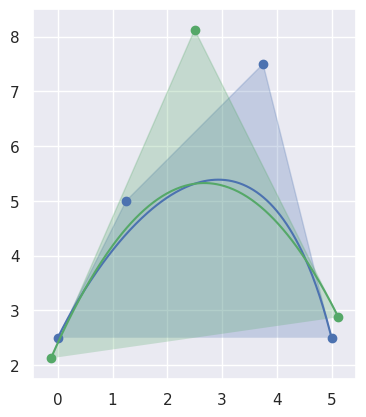>>> nodes = np.asfortranarray([
...     [0.0, 1.25, 3.75, 5.0],
...     [2.5, 5.0 , 7.5 , 2.5],
... ])
>>> curve = bezier.Curve(nodes, degree=3)
>>> reduced = curve.reduce_()
>>> reduced
<Curve (degree=2, dimension=2)>
>>> reduced.nodes
array([[-0.125,  2.5  ,  5.125],
[ 2.125,  8.125,  2.875]])

Returns

The degree-reduced curve.

Return type

Curve

specialize(start, end)

Specialize the curve to a given sub-interval.>>> nodes = np.asfortranarray([
...     [0.0, 0.5, 1.0],
...     [0.0, 1.0, 0.0],
... ])
>>> curve = bezier.Curve(nodes, degree=2)
>>> new_curve = curve.specialize(-0.25, 0.75)
>>> new_curve.nodes
array([[-0.25 ,  0.25 ,  0.75 ],
[-0.625,  0.875,  0.375]])


This is generalized version of subdivide(), and can even match the output of that method:

>>> left, right = curve.subdivide()
>>> also_left = curve.specialize(0.0, 0.5)
>>> np.all(also_left.nodes == left.nodes)
True
>>> also_right = curve.specialize(0.5, 1.0)
>>> np.all(also_right.nodes == right.nodes)
True

Parameters
• start (float) – The start point of the interval we are specializing to.

• end (float) – The end point of the interval we are specializing to.

Returns

The newly-specialized curve.

Return type

Curve

locate(point)

Find a point on the current curve.

Solves for $$s$$ in $$B(s) = p$$.

This method acts as a (partial) inverse to evaluate().

Note

A unique solution is only guaranteed if the current curve has no self-intersections. This code assumes, but doesn’t check, that this is true.>>> nodes = np.asfortranarray([
...     [0.0, -1.0, 1.0, -0.75 ],
...     [2.0,  0.0, 1.0,  1.625],
... ])
>>> curve = bezier.Curve(nodes, degree=3)
>>> point1 = np.asfortranarray([
...     [-0.09375 ],
...     [ 0.828125],
... ])
>>> curve.locate(point1)
0.5
>>> point2 = np.asfortranarray([
...     [0.0],
...     [1.5],
... ])
>>> curve.locate(point2) is None
True
>>> point3 = np.asfortranarray([
...     [-0.25 ],
...     [ 1.375],
... ])
>>> curve.locate(point3) is None
Traceback (most recent call last):
...
ValueError: Parameters not close enough to one another

Parameters

point (numpy.ndarray) – A (D x 1) point on the curve, where $$D$$ is the dimension of the curve.

Returns

The parameter value ($$s$$) corresponding to point or None if the point is not on the curve.

Return type
Raises

ValueError – If the dimension of the point doesn’t match the dimension of the current curve.

to_symbolic()

Convert to a SymPy matrix representing $$B(s)$$.

Note

This method requires SymPy.

>>> nodes = np.asfortranarray([
...     [0.0, -1.0, 1.0, -0.75 ],
...     [2.0,  0.0, 1.0,  1.625],
... ])
>>> curve = bezier.Curve(nodes, degree=3)
>>> curve.to_symbolic()
Matrix([
[               -3*s*(3*s - 2)**2/4],
[-(27*s**3 - 72*s**2 + 48*s - 16)/8]])

Returns

The curve $$B(s)$$.

Return type

sympy.Matrix

implicitize()

Implicitize the curve .

Note

This method requires SymPy.

>>> nodes = np.asfortranarray([
...     [0.0, 1.0, 1.0],
...     [2.0, 0.0, 1.0],
... ])
>>> curve = bezier.Curve(nodes, degree=2)
>>> curve.implicitize()
9*x**2 + 6*x*y - 20*x + y**2 - 8*y + 12

Returns

The function that defines the curve in $$\mathbf{R}^2$$ via $$f(x, y) = 0$$.

Return type

sympy.Expr

Raises

ValueError – If the curve’s dimension is not 2.

property degree

The degree of the current shape.

Type

int

property dimension

The dimension that the shape lives in.

For example, if the shape lives in $$\mathbf{R}^3$$, then the dimension is 3.

Type

int

property nodes

The nodes that define the current shape.

Type

numpy.ndarray